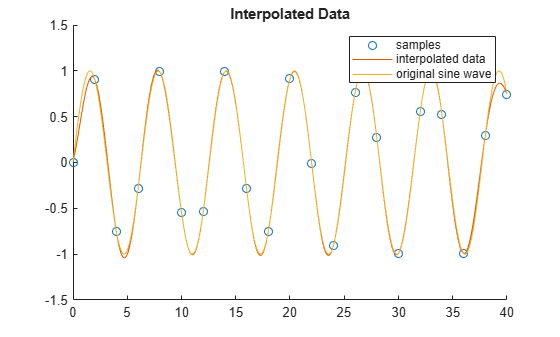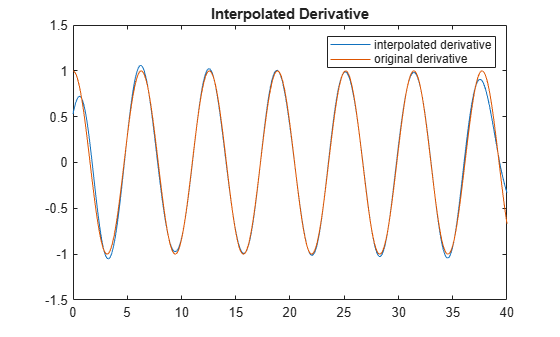lowpassResample

Convert signal from one sample time to another

Description

example

[vq,vdq] = lowpassResample(t,v,tq,config) returns an interpolated vector of samples vq and their derivatives vdq from input sample times t, input sample values v, and output sample times tq. The interpolation process is defined by the parameters in config.

Examples

collapse all

Sample a sine wave at pi samples per cycle.

t = (0:20)*2;
v = sin(t);

Define the interpolation sample times.

tq = (0:400)*0.1;

Define the interpolation configuration.

config.OutputRiseFall = 2; %Fixed step sample interval
config.NDelay = 5;
config.SampleMode = 'fixed';
config.CausalMode = 'off';

Perform the interpolation.

[vq,vdq]=lowpassResample(t,v,tq,config);

Scatter plot the samples, plot the interpolated data, and plot the original sine wave.

scatter(t,v);
hold on;
plot(tq,vq);
plot(tq,sin(tq));
hold off;
title('Interpolated Data');
legend('samples','interpolated data','original sine wave');Plot the interpolated derivative and the original derivative.

plot(tq,vdq);
hold on;
plot(tq,cos(tq));
hold off;
title('Interpolated Derivative');
legend('interpolated derivative','original derivative');Input Arguments

collapse all

Input sample times, specified as a fixed-step or variable-step vector.

Data Types: single | double | int8 | int16 | int32 | uint8 | uint16 | uint32 | logical

Input sample values corresponding to the input sample times defined in t, specified as a vector.

Data Types: single | double

Output sample times, specified as a fixed-step or variable-step vector.

Data Types: single | double

Interpolation parameters, specified as a structure with fields.

FieldDescriptionValueDefault
OutputRiseFallThe 0%–100% rise/fall time of the interpolated output.Positive real scalar1e-10
NDelayNumber of rise/fall times by which the interpolated output will be delayed with respect to the input.Positive real integer1
SampleModeInput sampling mode, either fixed-step discrete time or variable-step discrete time.fixed, variablevariable
CausalModeDetermine whether you want the interpolation process to introduce delay. Select CausalMode to introduce enough delay between input and output samples in the interpolation process so that the process is strictly causal.off, onoff

Data Types: struct

Output Arguments

collapse all

Interpolated samples, returned as a vector.

Data Types: single | double

Derivative of interpolated samples corresponding to vq, returned as a vector.

Data Types: single | double

collapse all

Delay in Interpolated Output

You can define the number of rise/fall times by which the interpolated output will be delayed with respect to the input using the config.NDelay parameter.

The default value of config.NDelay of 1 produces an interpolation that has no ringing due to the Gibbs phenomenon and also has modest rejected out of band numerical artifacts.

Setting config.NDelay to 5 introduces enough anti-aliasing filtering to satisfy most applications, but introduces ringing due to the Gibbs phenomenon.

Setting config.NDelay to 10 introduces enough anti-aliasing filtering to satisfy demanding applications, but at the cost of additional delay and computation.

Setting config.NDelay to greater than 10 is supported, but is not required normally.

Input Sampling Mode

You can define the input sampling mode using the config.SampleModeparameter.

The default value of config.SampleMode of variable assumes that the input values are the result of a zero order hold process. In this case, the signal value is always equal to the value of the most recent sample. This choice is appropriate for saturated signals for which the transition times are the most important consideration.

Setting 'config.SampleMode to fixed assumes that the input values are instantaneous samples of a mathematically continuous signal at uniformly spaced sample times. Use this option for signals that are subject to a significant amount of analog filtering.

Causality of Interpolation Process

You can define the interpolation process as causal or not using the config.SampleMode parameter.

The default value of config.CausalMode of off aligns the time scale of the interpolation with the time scale of the input. This is appropriate when all necessary input values are available in a single vector.

Setting config.CausalMode to on introduces enough delay so that the sample values are available before performing an interpolation. The interpolated sample times are therefore always delayed by a constant value with respect to the input sample times. The required additional delay in this mode is config.OutputRiseFall times config.NDelay. This behavior mimics the behavior of the Lowpass Resampler block.

Extended Capabilities

C/C++ Code GenerationGenerate C and C++ code using MATLAB® Coder™.